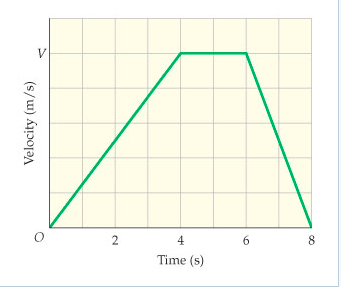# Problem: A car in stop-and-go traffic starts at rest, moves forward 21 m in 8.0 s, then comes to rest again. The velocity-versus-time plot for this car is given in the figure.A) What distance does the car cover in the first 4.0 seconds of its motion?B) What distance does the car cover in the last 2.0 seconds of its motion?C) What is the constant speed V that characterizes the middle portion of its motion?

###### FREE Expert Solution

Distance x(t) is given by:

$\overline{){\mathbf{x}}{\mathbf{\left(}}{\mathbf{t}}{\mathbf{\right)}}{\mathbf{=}}{{\mathbf{\int }}}_{{\mathbf{t}}_{\mathbf{1}}}^{{\mathbf{t}}_{\mathbf{2}}}{\mathbf{v}}{\mathbf{\left(}}{\mathbf{t}}{\mathbf{\right)}}{\mathbf{d}}{\mathbf{t}}}$

This implies that the distance is the area under the curve.

To determine the average velocity, we proceed as follows:

x = area under the curve.

For a trapezium, A = (1/2)(a + b)h = (1/2)(8 + 2)v

21 = 5v

v = 21/5 = 4.2 m/s

A)

In the first 4.0s, the distance covered is:

82% (284 ratings)###### Problem Details

A car in stop-and-go traffic starts at rest, moves forward 21 m in 8.0 s, then comes to rest again. The velocity-versus-time plot for this car is given in the figure.A) What distance does the car cover in the first 4.0 seconds of its motion?

B) What distance does the car cover in the last 2.0 seconds of its motion?

C) What is the constant speed V that characterizes the middle portion of its motion?